- DSP log - http://www.dsplog.com -

Need for I-Q modulator and demodulator

Posted By Krishna Sankar On April 5, 2008 @ 7:07 am In Analog | 40 Comments

Some time back, a friend mentioned that he is yet to understand the need for having both in-phase (I) and quadrature-phase (Q) signals in typical wireless systems.

In this post, the attempt is to bring out the motivation for having I-Q modulation and present the block diagram of a simple I-Q modulator (and demodulator).

I am using the text provided in Sec 5.2.1 of [DIG-COMM-BARRY-LEE-MESSERSCHMITT]as reference.

## Baseband PAM transmission

Consider a simple baseband transmission where the information is sent by modulating a pulse. This can be represented as

, where

is the symbol period,

is the symbol to transmit,

is the transmit filter,

is thesymbol index and

is the output waveform.

Pictorially the same can be represented as,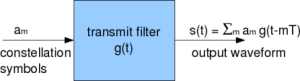Figure: Baseband PAM transmission (Refer: Figure 5.1 [DIG-COMM-BARRY-LEE-MESSERSCHMITT] ]

To transmit undistorted through the channel, the minimum bandwidth required is half the symbol rate (per the Nyquist criterion).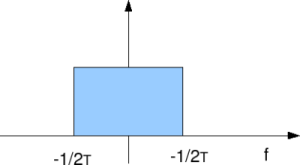Figure: Minimum bandwidth required for transmitting baseband PAM with symbol rate

Assuming that there are alphabets in , the spectral efficiency for basedband PAM is,

bits/second/Hertz.

BB-PAM : Baseband PAM

## Passband PAM transmission

Now, consider that the baseband PAM signal is upconverted to a carrier frequency by multiplication by a carrier, i.e.

.

The spectrum of the passband PAM is as shown below,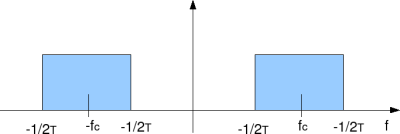Figure: Spectrum of passband PAM

To avoid transmit undistorted through the channel, we require a passband filter having bandwidth .

Assuming that there are alphabets in , the spectral efficiency for passband PAM is,

bits/second/Hertz.

PB-PAM : PassBand PAM

Can see that spectral efficiency of passband PAM is half of baseband PAM.

Note:

Passband PAM is composed of real signals and real signals have symmetric spectra. So, half the bandwidth is not carrying any ‘extra’ information.

## Moving to passband QAM

It is known that if a sine wave and a cosine wave is periodic over time , then they are orthogonal i.e.
.

Given so, a popular way which people came across to improve the efficiency of the passband PAM is to send information on the sine wave also in parallel, i.e.

.

This forms the passband QAM modulation. This signal requires the same bandwidth as passband PAM, however carries twice the informtion. For notational convineance, the above signal can be represented mathematically as,

, where

and

.

This forms the I-Q modulator circuit.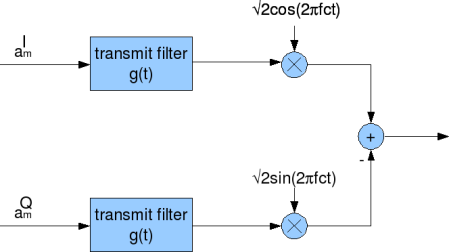Figure: IQ modulator

The IQ demodulator can be visualized as shown in the figure below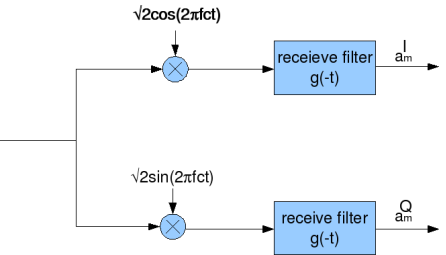Figure: IQ demodulator

On the combined signal, downconvert the cosine (inphase, I) and the sine (quadrature Q) arms, then proceed to do independent demodulation on each arm.

Summarizing,

(a) It is the need for improved spectral efficiency that resulted in I-Q modulation (and de-modulation).

(b) Another approach for improving the spectral efficiency of passband PAM is to filter away half the bandwidth (which is not carrying any ‘extra’ information). This is called Single Sideband Modulation (SSB).

Anyhow, it seems that using I-Q modulation is simpler to implement than circuit for filtering away half the spectrum. So, I-Q modulation stays.Reference

[DIG-COMM-BARRY-LEE-MESSERSCHMITT]Digital Communication: Third Edition, by John R. Barry, Edward A. Lee, David G. MesserschmittThanks,
Krishna